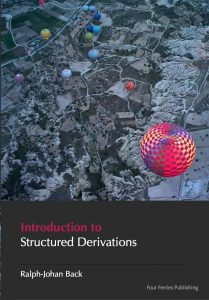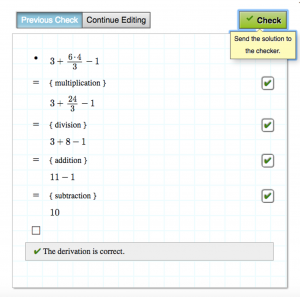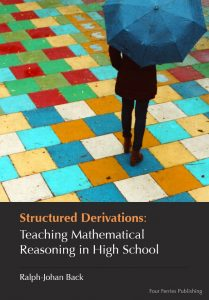# Education Material# Teacher Education in Math

### Learn to teach with Structured Derivations

Learn how to teach using Structured Derivations. There is a free tutorial on Structured Derivations that you can download as a pdf. There is also a free tutorial in the form of a digital textbook in eMath Studio.### Use eMath textbooks in High School Math

The eMath textbook series is developed by Math teachers from Finland, Sweden and Estonia. The books follow a teaching approach that uses the Structured Derivations methodology, where the idea is that every step is explained in words. This makes it easy to follow the examples that explain the theory. At the same time the approach forces the student to reflect on what they are doing. The books also introduce the students to logic, a step often skipped in school, but without which mathematics becomes magic instead of reasonable and understandable.But it requires too much time to write down the word explanations! No, our tools are designed to help you write math smoothly and will get your and your students’ derivations flowing effortlessly in no time. More about the math tools:

Math Tools

Videos on how to write structured derivations:

Simple Middle School Math Problems

Word problemsStructured Derivations: Teaching Mathematical Reasoning in High school

This book is intended for mathematics teachers who want to try out the method  in practice in teaching. It shows how to structure a mathematical derivation so that it is easy to see the overall structure of the argument.### Textbooks on Structured Derivations

Four Ferries Publishing offers two textbooks on structured derivations. The first one gives an overall description of the method, and explains by a large number of examples how to use the method in practical education at high school level. The second book is a handbook on structured derivations,  covering both the method and its logical foundation. Both books are  intended for mathematics teachers on all levels of math education, to help them make their explanations easier to understand and follow.

Teaching Mathematics in the Digital Age with Structured Derivations

This book extends the first book with a more thorough treatment of the logic behind high school mathematics, as well as the logical basis of the method. It also goes further, presenting material that is directed for introductory level university mathematics.